Select Page

# Modular Numbers and Time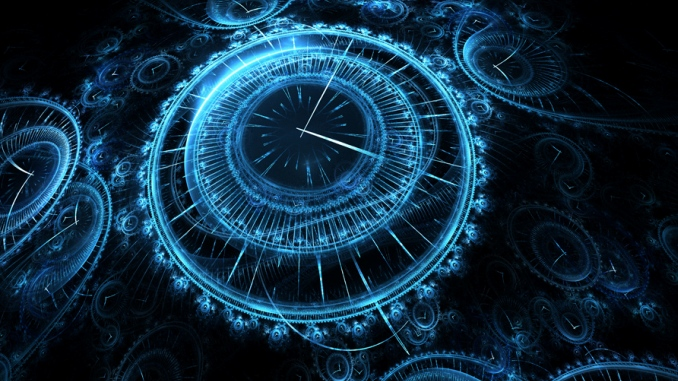In the exact same way that our clocks cycle around through 12 hours and start again with 1, modular mathematics cycles around and gives us the number of cycles plus a remainder or residue value. Likewise, we have put our planet on a 24 hour cycle, every cycle is 1 day plus how many hours it is depending on the time of day. The annual year is yet another cycle of 365.256 days, giving us 1 year, plus x amount of days depending on our calendar. This is a more natural cycle since the value for the amount of days is predicated on the rotating and revolving earth and not on an arbitrating splitting up of that day into so many hours. For anyone that has not been following this series from its inception, you can find the entire work here.

### Fibonacci Numbers and Remainders

There are endless wonderous facts about the Fibonacci numbers, many we have covered already. A few that are pertinent to our discussion today and their connection to Creation include the sum of the Fibonacci numbers through the 18th number in its sequence being 4180, which is analogous to the dynamic splitting of the Alef-bet we covered out the outset of this series.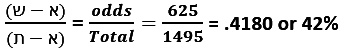Another pertinent fact is that the sum of the Fibonacci numbers through the 8th number in the sequence is 33, as in the 8 words/33 letters of Bereshit that comprise one of the 5 Essential Elements of Creation.  Meanwhile, the sum of the Fibonacci numbers through the 9th number is 54, as in the value associated with the 5th Essential Element of Creation, the Essential Cube of Creation

Also significant, considering this short initial section of the set of sequential numbers based on Phi(φ), is that the sum of the Fibonacci numbers through the 12th number is 232, as in the sum of the 4 aspects of the YHVH (יהוה), while the sum of the Fibonacci numbers through the 13th number is 376, as in the year and Spherical Time span 3760 and not coincidentally in the numerical value of Shalom(שלום), peace.

One of the many odd and wonderous properties of the Fibonacci numbers (0, 1, 1, 2, 3, 5, 8, 13…) is that the remainders of the sequences form repetitive patterns. It all has to do with how the digit fields are added together, Fn = Fn-1 + Fn-2, all starting from just the numbers 0 and 1.  In mod(10), which is just simple everyday base 10 mathematics, the final digits in the Fibonacci numbers (0, 1, 1, 2, 3, 5, 8, 13, 21, 34, 55…) will repeat every 60 numbers, like clockwork. Just like our arbitrary 60 minutes in an hour and 60 seconds in a minute. And all 60 of those final numbers add up to 280, as in Manzipach, the 5 final letters of the Alef-bet.

In mod(9) though, the cycle is every 24 numbers, as in the 24 hours in a day. Those 24 numbers will always add up to 99. In mod(8), the cycle is every 12 numbers, as in the 12 hours of our clock. Those 12 numbers will always add up to 32.

The sum of the repetitive cycles for the mods (7 – 10) is 112 cycles, as in the 112 Triplets of Creation; for mods (6 – 10) it is 137 cycles, as in the value of the word kabbalah, meaning “parallel” and “to receive,: and as in the Fine Structure Constant (1/137.0); and for all 10 mods, or mod(1- 10), it is 175 cycles, as in the 175 years of Abraham, the Patriarch.

Meanwhile, the sum of the digits, in all the cycles for all the numbers for all 10 mods (1- 10) is 585. Together, the complete value of the digits and cycles for all 10, as in the 10 sefirot (dimensions) is (175 + 585) = 760, which comes up over and over again as 3760 and 5760, and as 76.0, the square root of 5778, and the ordinal value of the Torah’s first word, Bereshit, “In the Beginning.”

Oddly, the sum of the cumulative sums (280, 280 + 99, …) of those 10 sets of the numbers from the remainders of the 10 mods (1- 10) of the Fibonacci numbers is 4949.

One oddity that seems to be the universe teasing us is that the final digit sequence from 13- 89 or …13, 21, 34, 55, 89… is 31459 as in Pi (3.14159). Some might say that since (5 + 5) = 10 in the number 55, the 10th Fibonacci number, we could theoretically add the missing 1 in 3.14159.  And as we just said, that seductive sequence will repeat every 60 numbers.

# The 27.5 HZ Frequency and Tesla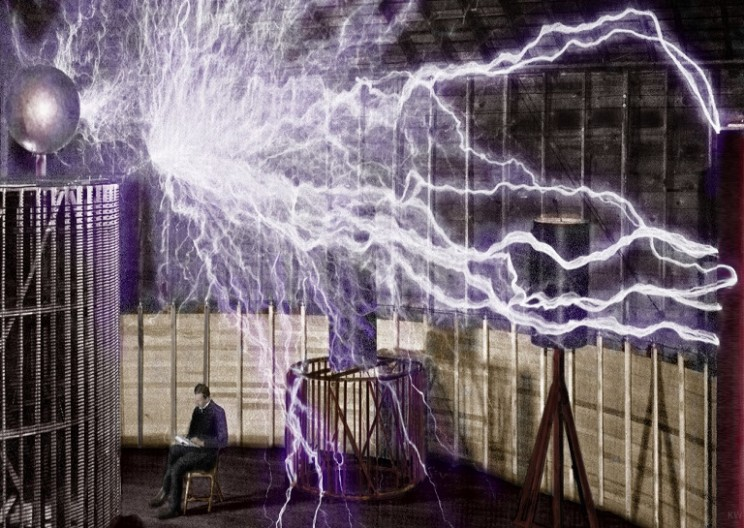Applying Mod (27.5) to the Fibonacci numbers has a repeat cycle of 20, which totals 165, as in the midpoint, 165 cubits, in each of the 4 bases of the Pyramid. None of this is accidental. None of this is coincidental.

Everything comes back to One (1).

There are exactly 58 or 390,625 words, letters and verses in the Torah.  In and of itself, that is a phenomenal sentence, which should make people get off Tik Tok and pick up a chumash.

Nevertheless, much like the Fibonacci sequence, the value 390,625, each one representing a different letter, word or verse in the Torah, began with 1 and then expanded because of 2.  The number One (1) divided by 2 = .5, then divided by 2 again and again 8 times gives us .00390625. This also words out to 1/256, the numerical value of the Ark of the Covenant. Using a little Tesla intuition, we can look at that repeated division not as an isolated series of mathematical operations but as a mathematical sequence or set (1, .5, .25, .125, ,0625, .03125, .015625, .0078125,  .00390625,…). Using Number Theory, or alternatively small gematria, this infinite set reduces to (1, 5, 7, 8, 4, 2, 1, 5, 7, 8, 4, 2…), an infinite repeat pattern of 157842. Besides the allusion to 5778 and 42, what we notice right off the top is that (1 + 5 + 7 + 8 + 4 + 2) = 27, as in the 27 positions of the Essential Cube of Creation and the corresponding 27 letters of the Alef-bet that the Torah is composed of. What takes a little more digging is that the sum of the reduced divisional sequence through .00390625 is (1, 5, 7, 8, 13, 11, 19, 23, 25) = 112, as in the 112 Triplets of Creation. If we limit this process to only the first 1, 5, 7, 8, 4, 2 repeat sequence, the total is (1, 5, 7, 8, 13, 11) = 45.

None of this is accidental. None of this is coincidental.

As we have previously explained, the numerical values of the 27 letters of the Alef-bet are based on a simple exponential equation and a sine wave that rides astride it, yet they are also 3 parallel groups of 9: 1 – 9, 10 – 90 and 100 – 900. It is because of that structure that the 27 letters sum to 4995. There is another way to get to 4995 though, and it involves the uniqueness of the sacred cubit and frequency (27.5”).

Tesla was obsessed with the number 3 and subsequently with 9 and 18.  He was also obsessed with frequencies. So were the kabbalists.  Let us think of 27.5 as a frequency, 27.5 Hz perhaps. It is also a very special number, unique in how it interacts with the number 9. As a basic mathematical principle or rule we can divide any number by another number, except zero that does not exist except as a placeholder.  Decimal places within numbers are just multiples or divisions of 10x so we can basically ignore them.  So, considering the set of all whole numbers individually as our numerator, we can divide any of them by another whole number (the denominator).  For arguments sake, let us extend that to one decimal place, starting with 1, 1.1, 1.2…, even though we know that is really the same as 10, 11, 12.  What we find is an endless stream of results for 1/1. 1/1.1, 1/1.2…. 2/1, 2/1.1, 2/1.2….3/1, 3/1.1, 3/1.2….

Some of those fractions result in whole numbers like 4/2 = 2, or in exact terminating numbers such as 1/1.6 = .625. Some of those fraction results are irrational numbers, an endless string of seemingly random digits. Others result in repeated patterns of single numbers, like .11111…. or .444444…. Some have triple repeat patterns like 11/2.7 = 4.074074…. For this exercise, let us ignore what comes before the repeating begins because that is a function of the size of the number. At a certain point, unless the result is irrational, all the numbers will settle into a repeat pattern. Many of the numbers will result in a 2-digit repeating pattern, such as .242424 or .424242 or .151515. Yet only a tiny set will result in 2-digit repeats that sum to 9, as in .090909…, .181818…, .272727…, .363636…, .454545…, .545454…, .636363…, .727272…, .818181… and .909090….

Before we begin analyzing this, we need to point out that the sum of .090, .181, .272, .363, .454, .545, .636, .727, .818, and .909 equals 4.995, as in the sum of the 27 letters of the Alef-bet.  If the decimal place is extended indefinitely as happens naturally, the result is 4.9999….. = 5, the number at the core of the Torah (58) and of Phi(φ) based on the square root of 5. As Tesla would ask, what generates this, what frequency in the universe generates these strings of 9 in the results of pure numbers?

As it turns out, there are only a few such numbers that can be used as denominators, the active part of the equation, to generate such results. They are 1.1, 2.2, 5.5. 11, 22, 27.5 and any multiple of 27.5 in the order of F(x) = 27.5(n + 2) At first glance that gives us exactly 8 frequencies through 110 and of them the number 22 stands out as reflective of the 22 Hebrew letters and the simple equation (27.55.5) = 22.   Yet on further reflection, we realize that F(x) = 27.5(n + 2) yields 55, 110, 137.5, 220, 275…, meaning that 1.1, 2.2, 5.5. 11, 22 are just derivative of 55, 110, and 220. Therefore, it is only by dividing a number by 27.5 or certain of its even number derivatives that we can generate strings of numbers with repeating sets of 9’s.

How interesting that the measure of the sacred cubit (27.5”) is a number that is mathematically unique amongst all other numbers, and that it is integrally connected to the number 9. Moreover, as we just saw, that makes it integral to the design and make of the Alef-bet, Torah, and Phi(φ).

Not every number that we divide by 27.5 will yields these special results based on 9.  The exception are the numbers that are derivate of 27.5 such as 1.1, 2.2, 5.5. 11, 22, 27.5, and any multiple of them. These will naturally wind up being whole or terminal numbers.

As it turns out, each of these special derivative numbers are exactly 11 units apart. And every of grouping of the 10 results between them ends in a complete set of repeating .363636…, .727272…, .090909…, …,.454545…, .818181…, .181818…, .545454…, .909090…, .272727…,  .636363… in that exact order, forever.

For what it is worth these are self-organized into 5 concentric pairs of 99: (.36 + .63), (.72 + .27), (.09 + .90), and in the center (.81 + .18).  And in case you were wondering if there was some hidden pattern in these 10 endlessly repeating numbers that are responding to the frequency 27.5, there is: (+36, -63, +36, +36, -63, +36, +36, -63, +36) or simply (+36, +36, -63).

The universe ordered these numbers this way, not our spreadsheets and certainly not Tesla, and yet… When we apply the revelatory cipher of taking their square roots, this repetitive pattern shows some very interesting results for us and for Telsa himself.  The results are the same whether we use the square roots of 36 or .36, so for simplicity, let us use 36, 72, 90, 45, 81, 18, 54, 90, 27 and 63.  The results in order: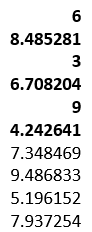The 3 – 6 – 9 results are highly recognizable from Tesla’s quote, “If you only knew the magnificence of the 3, 6 and 9, then you would have a key to the universe,” yet the 3 numbers they are interlaced with are highly significant to us and to the Kabbalists.

While the number 4.242641, as we have said many times, represents Moshiach Ben David (424), the YHVH (26) etc., the number 8.485281 is just that number doubled.

The 4th number of the repetitive set of 10 is 6.708203… and 6708 is the exact gematria value of the 42-letter Sword of Moses found in Exodus 15:11 with the chapter/verse coordinates 15 and 11 being indicative of the 15 Triplets and the 11 Triplets on one of the axes of the Alef(א) of Creation, as previously covered.  The 67 is also the numerical value of Binah.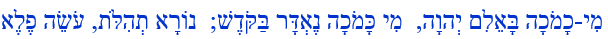The sum of 36, 72, 90, 45, 81, 18, 54, 90, 27 and 63 is 495, like the sum of the values of the Hebrew letters from Alef(א) to Zadi(צ). The rest of the values associated with Koof(ק) to Zadi sofit(ץ) total 4500.

Of those derivative frequencies (1.1, 2.2, 5.5. 11, 22, 27.5) of 27.5 that gave us the series of the resultant 9’s, the number 27.5 is the 6th one, as in the 6 handsbreadths that we have been told by definition equal 1 cubit.

The cycle of resultant 9’s are in sets of 10, falling in between every 11th derivative number, which matches our 10 and 11 sefirot (dimensional) model of the Tree-of-life, and also the string theory model of the universe.  While this repetitive pattern is endless, there is one distinct set of them that we can highlight.  The first numbers that result in the exact repetitions in ascending order from .0909 to .1818 through to .9090 form a special and unique set. They correspond to the numbers 25, 50, 75, 100, 125, 150, 175, 200, 225, and 250.  And the sum of the 10 repetitive 9 multiples work out to exactly 50, while the sum of the series above (25, 50…250) is 1375, recognizable as 50 cubits of 27.5 as in the 50 Gates of Binah and 50 cubit height of the Gates of the Holy Temple and of Noach’s Ark.

### The Frequency Built into the Pyramid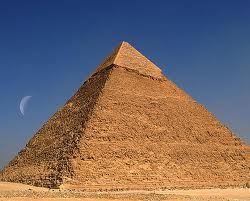The frequency emanates both ways, whether we divide by 27.5 or multiple it and that is exemplified in base of the Pyramid, 756.25’, which is 27.5 x 27.5.  It also spreads in all directions, as is exemplified by that same base, 330 cubits in that 330 = 12 x 27.5, or as explained in the Zohar, the light spreads inward and outward in all 6 directions of Zeir Anpin, 2 x 6 = 12.

The height of the Pyramid, 210 cubits, represents that expansion through time, 210 x 27.5 = 5775” or 5775 years. The time span that defines the Spherical Time equation, 5778 years when divided by the 27.5 frequency is 210.10909, the difference defined by the recurrent progression of the cubit’s 27.5 inches or (27.5 + 2.75 – .275 + .0275 – .00275 + .000275…)

As covered previously, the 210-cubit height of the pyramid which is 5775” or (5778 3) is equivalent to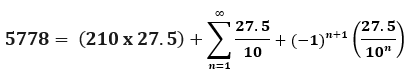The Pyramid had to be exactly 210 levels and 210 cubits high and the Israelites had to have lived in Egypt for 210 years or the sacred cubit may have been lost forever.  This leaves us with the possibility that the missing 3 inches from the limits from the Spherical Time paradigm was also not to be lost to us. As in the equation:The number 3 is entirely calculated as a limit of the frequency 27.5. As we ponder the length of the event horizon of the Spherical Time bubble perhaps this was a clue built into the fabric of the universe that was not meant to be lost either. Perhaps this was one of the reasons Tesla insisted on doing everything in 3’s.  The kabbalists had a different reason for doing everything in 3’s, but perhaps frequency and vibration was at the essence of both.

There is yet another connection between the sacred cubit/frequency (27.5) and Phi(φ). The growth rate of the random recurrent Fibonacci sequence is equal to 1.1319882487943, known as Viswanath’s constant, a limit on the bifurcations in chaos theory, in other words injecting order into chaos.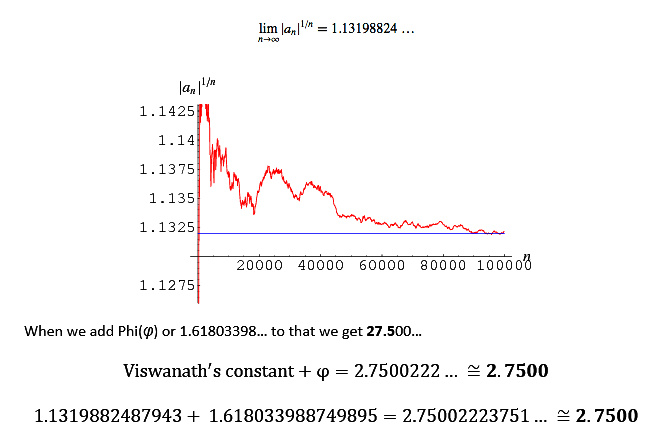As covered extensively, the 210 cubits is the exact height of the Tower of Truth (Emet) whose cubic volume is 44,100 cubits3 and 44,100/27.5 = 1603.6363 = 1600 and 363, or metaphorically H’Moshiach at 1600.  It was the 20 stacked cubes of the Tower of Truth that gave us the simple equation whose result equaled the mod9 values for the 18 sequential Fibonacci numbers that gave us the repetitive sequence 173553719826446289, which was also built into the repetitive layering of the digits 1 – 9.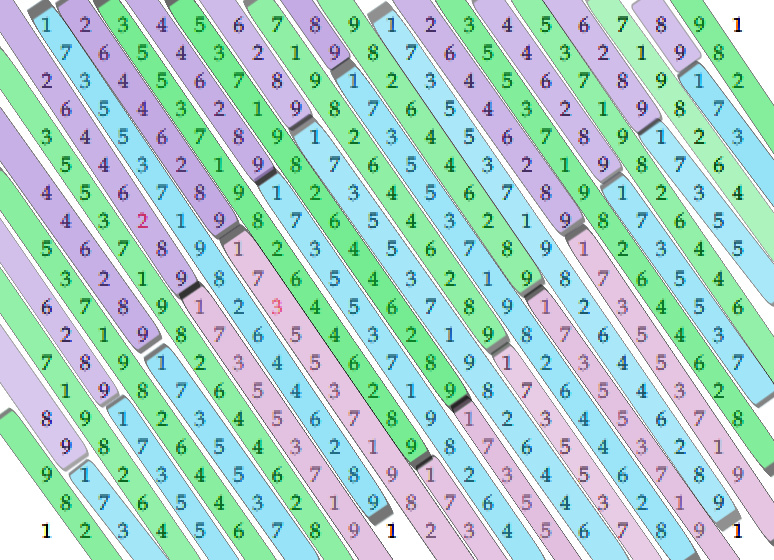Once again, 3’s and 9’s and the frequency of 27.5. While the diagonals in one direction give us the amazing and mysterious the repetitive sequence 173553719826446289, the diagonals in the other direction (181818, 272727, 363636, 454545, 545454, 636363, 727272, 818181, 999999) give us the remainders when we divide by 27.5.  Thus, we see the frequency of 27.5 interacting with the simple digits 19 yet again in the simplest and most wondrous natural ways.

### Another Interaction of Spherical Time Markers and the 27.5 Frequency

That 1600 years derived from the Tower of Truth is also its base (400 sq cu) times 4. It is also 56 years shy of the year of the Flood, 1656, whose anniversary is this Election Night. Given the Divine Calendar and Spherical Time, the year 5708 HC, when Israel became a nation, coincides with 1948 CE. Given what we just discussed about the Pyramid’s enduring measurements, it is most interesting that the sum of (5708 x 27.5) and (1948 x 27.5) = 210540. The 210 cubit height is a most obvious analogue, quickly followed by the (210 cu height plus the 330 cubits base) equaling 540, a number most associated with the Essential Cube of Creation.

Another time equation that could only be understood in the hyperspace modality that the kabbalists call Binah is that this monumental simultaneous conjuncture in Spherical Time, (5708 + 1948) less the year of the Flood, (5708 + 1948) – 1656 = 6000, as in the 6th millennium, the common prophetic time span for the final redemption. This leads us back to the final dimension of the Pyramid, its apothem, 612’, which is the gematria of Covenant(Brit). When divided by 27.5, the sacred frequency of 612/27.5 equals 22.25454, as in (6000222) = 5778.

Nonetheless, referring to 210,540, and “the writing is on the wall,” one of the revelations pointed out earlier in this series is that the complete value of its initials, is 540.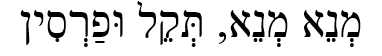One other alignment that we pointed out earlier in this series, which will be critically important to everyone by the end of this chapter, is that the product of the first 6 digits in Pi are (3 x 1 x 4 x 1 x 5 x 9) = 540. Keep in mind that all this is referring to a particular expansion of the sacred frequency 27.5 HZ. as a resonance and marker in time.

An apropos reminder of what was detailed earlier in this series, though it has little to do with what will be revealed later in this chapter, is that the next 6 digits in Pi, (2 x 6 x 5 x 3 x 5 x 8) = 7200, and that our trusty ancient gematria cipher reveals that (2 x 6 x 5 x 3 x 5 x 8)/(2 + 6 + 5 + 3 + 5 + 8) = 248.2758621… Thus representing the sacred frequency (27.5) along with the 248 dimensions of the E8 Lattice; the numerical value of Abraham(248); the 248 columns in the Torah matrix; the precise 248,000(φ) words, letters, verses, columns and rows in the Torah; and so much more.

Considering the relationship of the sum of 27.5(5708 + 1948) = 210540 to ((5708 + 1948) – 1656) = 6000, it is more than interesting that (5708 x 27.5) + (1948 x 27.5) – (1656 x 27.5) or 27.5(5708 + 1948 1656) = 165,000, as in the 165 cubits to the score line where the apothem (covenant) is in the middle of the base of the Pyramid. Is this reflective of the Covenant of Halves between Abraham and the Creator in 2018 HC? After all, the Covenant of Halves is found in parsha Lech-Lecha, the only portion in the Torah named with a doubled word. The word value is 5050.

Speaking of doubles, there is one other note, considering that the Pyramid was built utilizing the 27.5” cubit and that it has a double thick layer of stone at its 35th level. We have previously discussed that this was analogous to the 35th verse of the Torah where we find the first double Name YHVH Elohim (אלהים יהוה) of G-d, whose numerical value 112 matches the 112 Triplets.  When we divide 35/27.5, we get 1.272727… or 1.273, the universal harmonic, which was also the distance from Moses death (2488) to the spherical year 3760. This is the same harmonic number that is built into the relationship of the Earth, Moon and Sun and that squares the circle.  It is also the built into the 401,273 words, letters, verses, columns and rows in the Torah, which when divide by Phi(φ) equals 248,000. Furthermore, it is the same universal harmonic that when multiplied by the cube root of 27.5 equals 3842, the value of the first 42 letters of the Torah: 1273 327.5 = 3842.

# The 27.5 HZ Frequency Determines the Speed of Light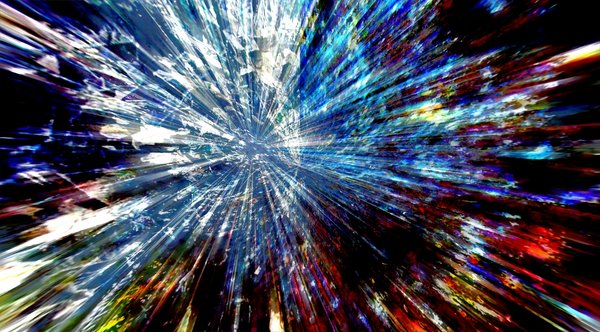In our physics, a frequency is the distance between two wave peaks and the higher the frequency the closer together those peaks and thus the shorter the distance. That pretty much defines the entire electromagnet scale as we understand it. If 27.5 was a frequency, say 27.5 HZ, its corresponding wavelength in space, meaning that it is solely contingent on the speed of light, would be 6773.90539 miles. A single wavelength would take up most of the diameter of the Earth. The combination of the Binah (67) and Chochma (73) values is easily recognizable, but this could be entirely coincidental. This means that the speed of light/1 cubit, or 186,282 mps/27.5 = 6773.9.  What is not coincidental is that 6773.90539 miles is certainly 6774 miles and that the sum of the 10 square roots of the resultant 10 remainders from .090909 to .909090 is 6.774

The length of the wave created by the frequency 27.5 is also the sum of the square roots of its 10 digital residues. There should be no logical correlation between them unless those 10 residues relate to the speed of light, which makes no scientific sense, unless the universe was intentionally designed that way.

In order to evaluate whether this is a causal relationship or not, we need to break down the mathematical process. So breaking it down, the wavelength of frequency 27.5 Hz based on the speed of light is equivalent to the sum of the square roots from (1 – 10) + 10(square root of .090909…), knowing that the sum of the numbers (1 -10) = 2 x 27.5.  Breaking that down even further we see that the square root of .909090 = 3 x the square root of .010101, and we already know that 3 can be derived from a recurrent progression of 27.5.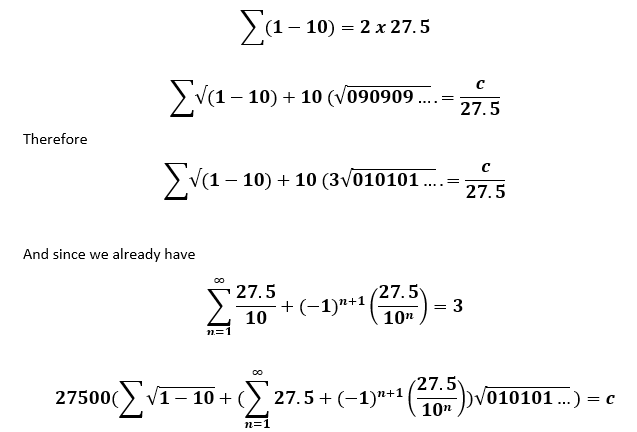I realize that looks complex, but what it means is that the speed of light, known as c in scientific terminology, and measured as about 186,282 miles per second is entirely based on the simple measure of the sacred cubit and frequency, 27.5, and the simple numbers 110, especially 1 and 10.

Pretty special number that 27.5 is.  There is almost no aspect of our modern physics that is not dependent on the constant (c) for the speed of light. And yet, the universe was created so that it was based not on propagating waves or imaginary particles but on a single simple number, 27.5 and the simplest of base 10 mathematics, what we have previously studied as the 10 fields from 1 to 10.

As we just saw the speed of light, 186,282 mps (miles per second), ties the sacred cubit/frequency (27.5) to the essence of the number 9 throughout as delineated by the 10 sefirot (dimensions). This is the same sacred cubit that is dedicated to be used in the building of the Future Holy Temple. It is the same concept of frequency at the core of Spherical Time.

Is that really so surprising? Actually it is, depending on your level of certainty. To 99.9999999999% of the population it would be shocking. We, those of us who understand or are trying to, keep asking why 5778 years, and why would it be split into 3760 years and 2018 years. And moreover, why does this Spherical Time bubble have to conform to these parameters?  If it has to do with anything it would be light as a measure of time. So how surprising or shocking is it that the ratio of 3760/2018 = 1.86282, which is 1/100,000 of the speed of light, 186,282 mps.  The exact ratio would be 3760/2018.45, so perhaps the number 45 that keeps coming up with regards to Spherical Time has always played a part in the equation and the balance that decides what happens at the edge of the time bubble.

The speed of light is not the speed of a photon or even of a wave. It is an observable effect. It is also the differential separating, or tying together, two simultaneous bubbles of time.

# The 27.5 HZ Frequency and the 112 Triplets

If we apply the same division by 27.5 to the 112 Triplets at the core of our Creation we get some interesting results.  Examining only the cycles and not the remainders, which we already know will all be in the order of 9, as illustrated above, we see that the sum of the 14 Triplets of the 42-Letter Name of G-d is 133.3 (exactly 1/3 of 4) and that of the 15 Triplets of the Shema is 34.7, and that (133.3 + 34.7) = 168 or 4 x 42.

The sum of those cycles for the 11 Triplets of Bereshit is 107, as in the sum of all the integers from 1 – 107 = 5778, the surface temperature of the Sun, 5778 K, that produces that light described above.

When we add the cycles of all three of these sets of Triplets we get (133.3 + 34.7 + 107) equals 275, our sacred cubit and essential frequency.

The cycles for all 72 Triplets are an additional 315.8, giving the 4 sets of Triplets a total of 590.8 cycles. It also gives the 4 sets of Triplets a total of 207 for their remainders, with 207 being the numerical value of Light (אוֹר), also of secret (רז).  The actual sum of the 72 remainders of the 72 Triplets is 3303 in simple form (18+27) and 3336.3636… in extended form (.181818… + .272727…). It is just one more complex design element built into these 72 Triplets (3 letters) whose 216 square roots by letter and 72 by Triplet total 1000.0.

If we were to add the 5th Element, the sum of the 27 Letters in the cycle of (division by) 27.5 we would get 172.1 with a simple remainder or 45. This boosts the total for all 5 Elements to approximately 763, and it is at digit #763 in Pi where we find the numeric string …999999…, six (6) 9’s. The value 763 is also the value of the 9 Triplets of the 1st column of the 72 Triplets. Moreover, the total 763 is analogous to the sum of the cube roots (7.63) of all 10 of the resultant remainders from .090909 to .909090. Perhaps Tesla was on to something.

For what it is worth, if we were to take the 27.5 division of those 5 cycle values for the 5 Essential Elements they would total 27.74… and (67.7427.74) is 40.

# The Frequency of the Solar System.

We have already seen in great detail how the measures of the Earth, Sun and Moon are repeatedly related to one another through the universal harmonic 1.273 in terms of their radii, diameters, and distances apart. We have also seen how the ratio of the volume of the inscribed cube to the Sphere is 2.7202699 or 2.72027, which is (π√3)/2.

The Moon’s circumference, 6782 miles, is about 8 miles off the exact wavelength of the 27.5 frequency, the frequency of 1 cubit, 6774 miles.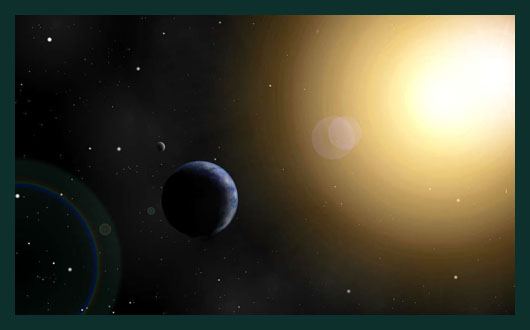The Earth’s diameter varies according to where it is measured from 7900 at the poles to 7926 at the equator. At 7920 miles, its circumference is 24881 miles, giving us the year Moses died (2488) again, as in 1273 years before 3760, but that is somewhat meaningless.  It is obviously several times larger than the Moon’s. It is 3.676 wavelengths of 27.5 Hz at the equator, or 6774 mi/.272022, which is exceeding close to 2.72027, the ratio of the volume of the inscribed cube to the Sphere, especially given the variation in the Earth’s diameter.

The Moon is a sphere approximately equal to the frequency of the sacred unit (27.5). The Earth is a sphere that circumscribes the moon as if were a cube.

The Sun has a circumference of 2,720,984 miles or roughly 2.72027 times 1 million (106) miles. The Sun is a sphere whose size is 1 million times the hyperspace (metaphorical) ratio between the Earth and the Moon. One million (106) is a 6-fold expansion of 10, as in the 10 sefirot(dimensions), a design that strictly corresponds to the 6 folded dimensions of string theory (the Calabi–Yau manifold) and the 6 bundled dimensions of Zeir Anpin, which is what the Kabbalists have always said that the Sun represents.

The harmony of the cosmos. And to think, our musical scale is set to the same intonation. Imagine if this could be harnessed!

Is there a reason the Hebrew word for peace (שלום) was given the gematria 376, about 3760 years before 3760, the edge of the dual time bubble?

# Inverses of the 10 Remainders

All 10 remainders were derived from a single cubit(27.5).  The pattern is a simple 1 – 10 – 1 – 10… or 10 consecutively out of every 11: 10 sefirot (dimensions) out of every 11. Think of it as a string of light and every 11th is turned off.  The 10 bright bulbs represent the complete set of 10 complementary infinite remainders based on the number 9. They will always add up to 5.  The 11th number will always be a simple finite number.

The inverse of the 4th remainder of those 10 is 1/.363636 = 2.75000… simply put, the sacred cubit (27.5 inches) and sacred frequency (27.5 Hz). Pretty fitting, as it is the residue most structurally connected to 3, 6, 9, and since 363 is the repeated gematria of H’Moshiach. The sacred cubit and frequency (27.5) and H’Moshiach (363) have an inverse relationship and thus when multiplied together (27.5 x .3636363), they give us One(1).

The inverses of the 1st, 2nd, 5th and 9th remainders are respectively, 11.0000, 5.50000, 2.20000, and 1.10000, all reflective of those numbers’ derivative relationships with 27.5 that we previously discussed. We could even say that they must be derivative of the 4th remainder that we just highlighted, 2.75.

The inverse of the 6th remainder, 1/.545454 = 1.833333…, analogous to both the numerical midpoint of the 42-Letter Name (1833) and the 1833 letter Samechs (ס) of numerical value 60 each found in the Torah.

The inverse of the 8th remainder, 1/.727272 = 1.37500…, is analogous to the 50 cubit height (1375”) of the 10 Gates of the Holy Temple and of Noach’s Ark.

The inverse of the 7th remainder, 1/.636363 = 1.571430, and while 1571 is the 248th prime number, it is also one-half of 3.142860.  The value 3142 has been covered repeatedly already as the small gematria of “I am that I am (אהיה־אשר־אהיה)” found at Exodus 3:14  It is also the total numerical value of the 3 iterations of the Names Ehyeh (אהיה)  and YHVH(יהוה). It is also the difference (3.1428) between the atomic weights of gold, silver, and copper(368.3988), associated with the central 2 Triplets of the 72 Names and the sideral year, 365.256. We find it again appropriately as 7.3142, the result of dividing the 42-Letter Name by its 1st verse, 3701/506 = 7.31422, and also by dividing 9 by that first verse in small gematria, 9/.123049 = 73.142 or more accurately, 73.141593

### The Incredible Equation

The inverse of the 3rd remainder, 1/.272727 = 3.666… or (51.333), which is interesting considering that the 27.5 division process yields 133.3 cycles of 27.5 in the total 14 Triplets of the 42-Letter Name.

Moreover, as discussed in chapter 26, the small gematria of the 42-Letter Name is 173, and 1/42 x 1/137 = .000173… The connection is that 42 times the Fine Structure Constant (1/137.0…) minus the Coupling Constant or (42/αg) = (42/137.03599.302822131), which equals .0036666… or 11/3000.

Of all the symmetries, indeed supersymmetries, that we have shown with regards to the sacred cubit (27.5”) and our universe, this next one would probably be regarded the most astounding by today’s physicists, though replacing the speed of light with the 27.5 Frequency is pretty special unto itself.

Through the simple division of the Fine Structure Constant by the sacred cubit (27.5) we get (1/137.03599)/27.5 = .00265358, as in Pi (3.14159265358…). We can align the digits simply by dividing the Fine Structure Constant by (27.5 x 102) or 100 cubits, which is the height of the Future Holy Temple. This allows for the equation Pi(π) – (1/137.03599)/27500 = 3.14195000000…., an exact 6-digit blank space or hole in Pi(π).

A slight modification in the calculation of the Fine Structure Constant as 1/137.035671756… would work out to exactly Pi. In other words, the difference between them would be exactly 3.14195. Do we have the right number for the Fine Structure Constant? Please note that the calculated value 1/137.0356+ was the prior established figure for the Fine Structure Constant until it was retooled just 2 years ago. It was ascertained with a degree of uncertainty, leaving 1/137.0356+ as extremely plausible, especially give the speed of light’s similar connection to 27.5.

## π – (1/137.035671756+)/27500 = 3.1419500000000000000 = 3.14159

In terms exclusive to Pi(π):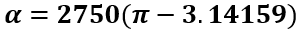There are several different ways to calculate the Fine Structure Constant, most of which includes calculating Pi, Planck’s constant, the speed of light, and the elementary charge. Some also include calculating the magnetic constant, electric constant and the coulomb constant. There are still other complicated ways: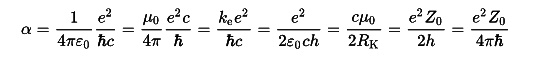Yet, all that is needed is a knowledge of Pi (π) and the sacred cubit:What this means is that several of these primal parameters might also be based on Pi(π) and the sacred cubit/frequency (27.5).  Wherever you see c in the above equations we can replace it with our speed of light equation and the Fine Structure Constant with the simple equation above to see what else shakes out.

When we divide both Pi(π) and Phi(φ) by the sacred cubit (27.5), or alternatively the sacred frequency (27.5 Hz) we get 0.114276096 and 0.0588376 respectively. Then when we add them and take their inverse the result is (1/0.173113696) = 5.77655, as in 5777 perhaps.

More thought needs to go into this as the Fine Structure Constant is based on Planck’s constant, which is a frequency and thus based on cycles of 4π or 2π, depending on whether we are talking about cycles or radians. As you may recall, the Spherical Time equation also relies on 4π or 2π and it is for a similar reason. More on that in subsequent posts; nevertheless, the Fine Structure Constant is a fixed number derived from Pi (π), and yet when multiplied by the sacred frequency (27.5) we find that it is part of Pi (π) itself. This is a conundrum for sure, for now.

This may be just kabbalistic alchemy, but 1/.00265358…. = 376.847997588 or 376.848 with 376 being the value for Shalom(שלום), peace and associated with 3760 years, and 848 being 2 x 424, Moshiach Ben David.  Maybe not, considering 358 is the numerical value of Moshiach and 265 is the fusion of the YHVH (יהוה) and Adonai (אדני), and 5358 is 420 years prior to 5778. Or that the 4 Triplets (314 + 159 + 265 + 358 + 4)/4 average 275.

In case it was not clear, the equation, 1/.00265358…. = 376.847997588, an obvious connection to Pi(π), is derived from (137.0356 x 27.5) = 3768.47997588.  What definitely was not clear and needs to be pointed out is that there are 79975 words in the Torah.  Apparently, the universe can walk and chew gum at the same time.

As for the 9th and final inverse to discuss, since .818181 is (3 x .272727) and since 1/.818181 = 1.22222, the result is the same 3.666 as in the inverse of the 3rd remainder, 1/.272727 = 3.666

This was a lot to take in. The sacred cubit is a unique measurement in our universe, and it is also a unique frequency that governs the Speed of Light, the cyclical dynamics of the integers, the Fine Structure Constant, and it integrates with Pi(π) and Phi(φ). As it all intertwines with our Spherical Time bubble, peace and Moshiach are in the offering, just as the Event Horizon is winding down.  We have never had more knowledge at our disposal. It is time to do something with it.

For anyone that has not been following this series from its inception, you can find the entire work here.